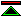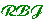# On the Building Blocks of Mathematical Logic

### by Moses Schönfinkel

This is the first published work in the field which came later to be known as combinatory logic.

Schönfinkel shows how the use of bound variables in logic can be dispensed with. The use of higher order functions makes possible the reduction of logic to a language consisting of one constructor (the application of a function to an argument) and three primitive constants U, C (now usually called K) and S. A function is termed "higher order" if it will accept a function as an argument, or return one as its result. U, C, and S are all higher order functions.

The meaning of these primitives may be understood through the following informal definitions:

Cxy = (C(x))(y) = x
C is a function which given any value x, returns the constant x valued function.
Sfgx = ((S(f))(g))(x) = (f(x))(g(x))
S is a function which combines two functions, say f and g, supplied as successive arguments. The resulting function, given a value x, returns the value obtained by applying the value of f at x to the value of g at x.
UPQ = (U(P))(Q) = forall x. not( P(x) and Q(x) )
U is a generalised scheffer stroke. It should be thought of as applying to two predicates and returns the universal quantification of the negated conjunction of the two predicates.

These combinators are sufficient to enable arbitrary first order predicates to be expressed without the use of bound variables, which appear in first order logic whenever a quantifier is used. This can be demonstrated most straightforwardly using a simple algorithm which converts lambda-expressions to combinators. They are not limited to first-order predicates, but without some constraints (equivalent to those found in first order logic, or more liberally and appropriately to those in Church's Simple Theory of Types [Church40]) the logic which results from the use of these combinators is at risk of proving inconsistent. Combinatory logic has traditionally tried to avoid type constraints and has therefore been dogged with difficulties in achieving strength and consistency.

Schönfinkel's paper remains an accessible introduction to combinatory logic which makes clear the original motivation for this innovation.©created 1995/12/9 modified 1998/12/10